# 可视化卷积神经网络的激活

### 加载预训练的网络和数据

`net = squeezenet;`

```im = imread('face.jpg'); imshow(im)``````imgSize = size(im); imgSize = imgSize(1:2);```

### 查看网络架构

`analyzeNetwork(net)`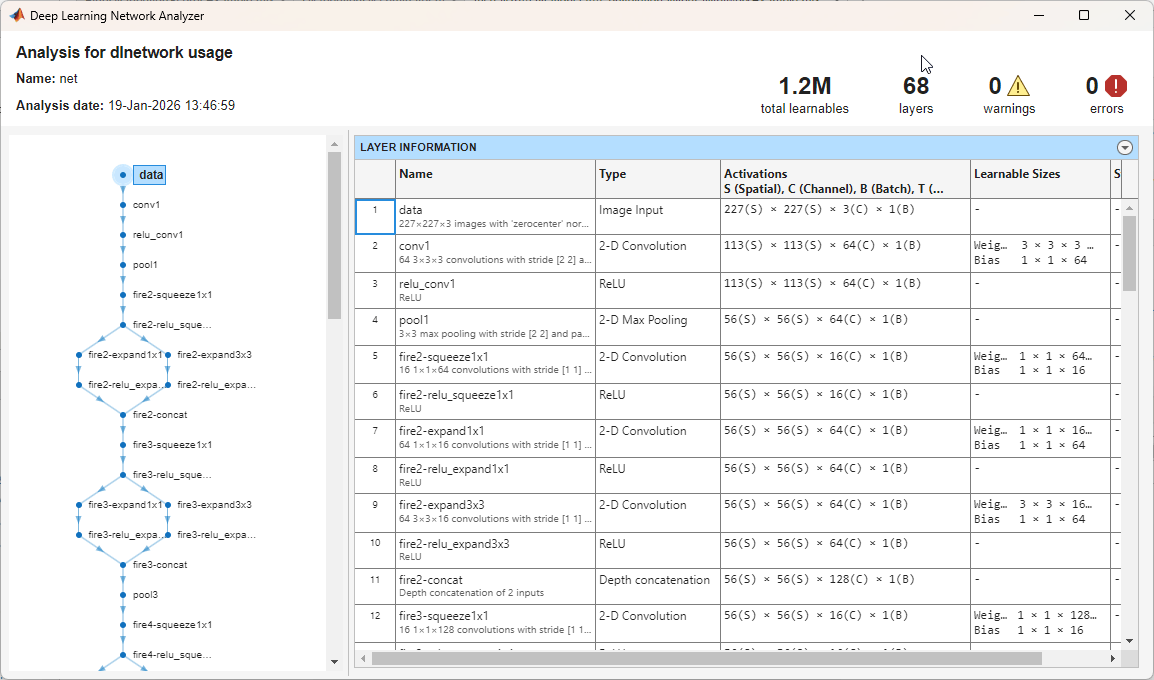### 显示第一个卷积层的激活

`act1 = activations(net,im,'conv1');`

```sz = size(act1); act1 = reshape(act1,[sz(1) sz(2) 1 sz(3)]);```

```I = imtile(mat2gray(act1),'GridSize',[8 8]); imshow(I)```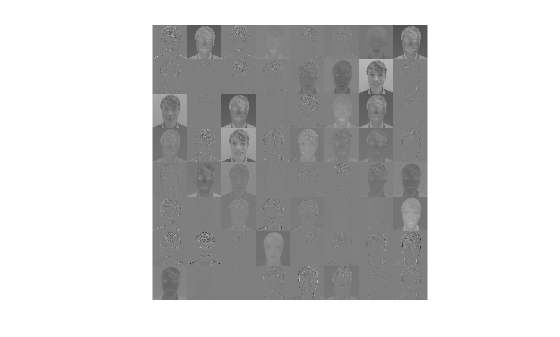### 调查特定通道中的激活

```act1ch22 = act1(:,:,:,22); act1ch22 = mat2gray(act1ch22); act1ch22 = imresize(act1ch22,imgSize); I = imtile({im,act1ch22}); imshow(I)```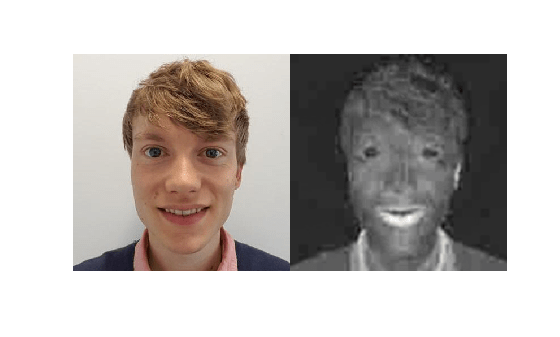### 查找最强的激活通道

```[maxValue,maxValueIndex] = max(max(max(act1))); act1chMax = act1(:,:,:,maxValueIndex); act1chMax = mat2gray(act1chMax); act1chMax = imresize(act1chMax,imgSize); I = imtile({im,act1chMax}); imshow(I)```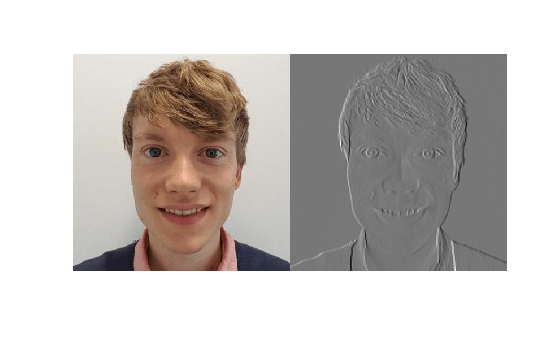### 调查更深的层

```act6 = activations(net,im,'fire6-squeeze1x1'); sz = size(act6); act6 = reshape(act6,[sz(1) sz(2) 1 sz(3)]); I = imtile(imresize(mat2gray(act6),[64 64]),'GridSize',[6 8]); imshow(I)```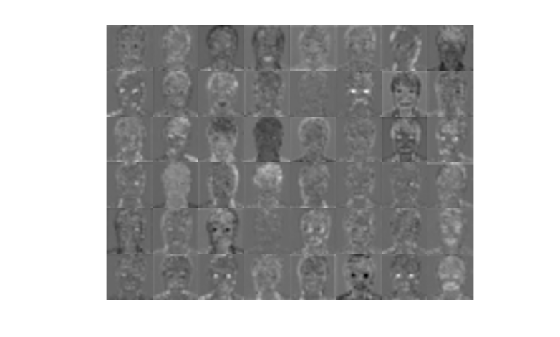```[maxValue6,maxValueIndex6] = max(max(max(act6))); act6chMax = act6(:,:,:,maxValueIndex6); imshow(imresize(mat2gray(act6chMax),imgSize))```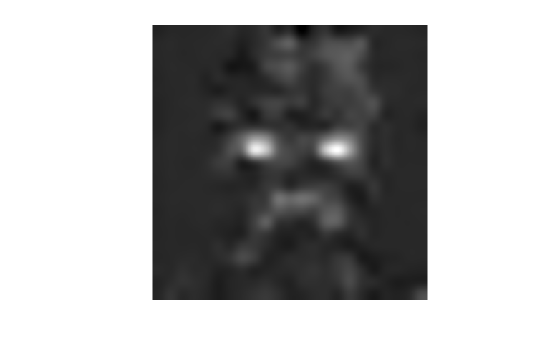```I = imtile(imresize(mat2gray(act6(:,:,:,[14 47])),imgSize)); imshow(I)```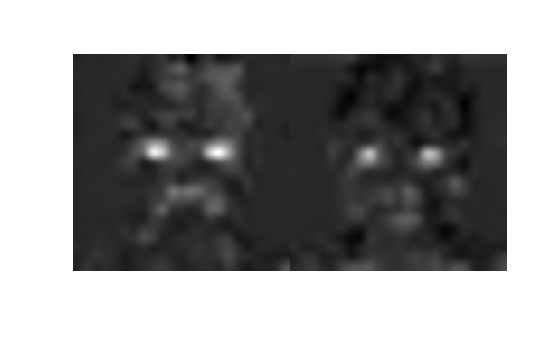```act6relu = activations(net,im,'fire6-relu_squeeze1x1'); sz = size(act6relu); act6relu = reshape(act6relu,[sz(1) sz(2) 1 sz(3)]); I = imtile(imresize(mat2gray(act6relu(:,:,:,[14 47])),imgSize)); imshow(I)```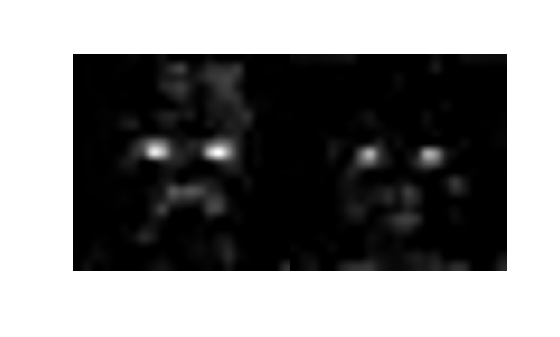`fire6-squeeze1x1` 层的激活相比，`fire6-relu_squeeze1x1` 层的激活清楚地定位到具有强面部特征的图像区域。

### 测试通道是否识别眼睛

```imClosed = imread('face-eye-closed.jpg'); imshow(imClosed)``````act6Closed = activations(net,imClosed,'fire6-relu_squeeze1x1'); sz = size(act6Closed); act6Closed = reshape(act6Closed,[sz(1),sz(2),1,sz(3)]);```

```channelsClosed = repmat(imresize(mat2gray(act6Closed(:,:,:,[14 47])),imgSize),[1 1 3]); channelsOpen = repmat(imresize(mat2gray(act6relu(:,:,:,[14 47])),imgSize),[1 1 3]); I = imtile(cat(4,im,channelsOpen*255,imClosed,channelsClosed*255)); imshow(I) title('Input Image, Channel 14, Channel 47');```